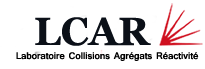# Laboratoire Collisions Agrégats Réactivité - UMR5589

Université Paul Sabatier - Bat. 3R1b4 - 118 route de Narbonne 31062 Toulouse Cedex 09, France

## Numerical generation of hyperspherical harmonics for tetra-atomic systems

A numerical generation method of hyperspherical harmonics for tetra-atomic systems, in terms of row-orthonormal hyperspherical coordinates—a hyper-radius and eight angles—is presented. The nine-dimensional coordinate space is split into three three-dimensional spaces, the physical rotation, kinematic rotation, and kinematic invariant spaces. The eight-angle principal-axes-of-inertia hyperspherical harmonics are expanded in Wigner rotation matrices for the physical and kinematic rotation angles. The remaining two-angle harmonics defined in kinematic invariant space are expanded in a basis of trigonometric functions, and the diagonalization of the kinetic energy operator in this basis provides highly accurate harmonics. This trigonometric basis is chosen to provide a mathematically exact and finite expansion for the harmonics. Individually, each basis function does not satisfy appropriate boundary conditions at the poles of the kinetic energy operator ; however, the numerically generated linear combination of these functions which constitutes the harmonic does. The size of this basis is minimized using the symmetries of the system, in particular, internal symmetries, involving different sets of coordinates in nine-dimensional space corresponding to the same physical configuration.

doi:10.1063/1.2218515

Voir en ligne : J. Chem. Phys., 125, 133505 (2006)